# Matrices: multiplication, rank, determinant, inverse and Rouche-Frobenius theorem

Classified in Mathematics

Written at onEnglish with a size of 155.53 KB.

System Types
The systems of equations can be
classified by the number of solutions that can arise. According to that case may have the following cases:
· Incompatible system if it has no solution.
· Compatible system if you have any solution in this case can also distinguish between:
or compatible system determined when it has a finite number of solutions.
indeterminate
or compatible system when it admits an infinite set of solutions.
Fitting and classification: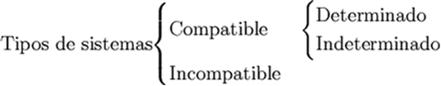Calculating the rank of a matrix for determining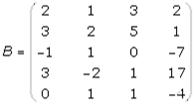1. We can rule a line if:.
· All the coefficients are zeros.
· There are two equal lines.
A line is proportional to another.
A line is a linear combination of others.
Delete the third column because it is a linear combination of the first two: c 3 = c 1 + c 2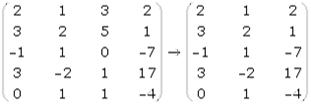2. We check if you have rank 1, for it must be satisfied that at least one array element is not zero and therefore its determinant is not zero.
| 2 | = 2 • 0

3. Will rank 2 if there is any square submatrix of order 2, such that its determinant is not zero.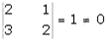4. Will rank 3 if there is a square submatrix of order 3, such that its determinant is not zero.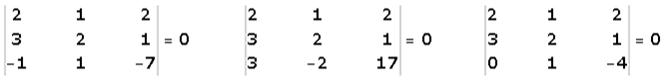As all the determinants of the submatrices are zero has rank 3, then r (B) = 2.
5. If you have rank 3 and there is a submatrix of order 4, whose determinant is not zero, you have rank 4. In this same way you work to check if you have range greater than 4.
Determinant
3x3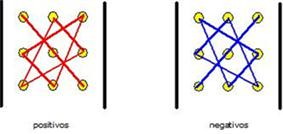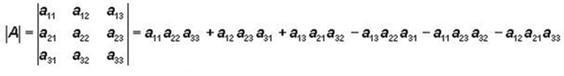Discussion of systems: Rouche-Frobenius theorem

The necessary and sufficient condition for a system of m equations and n unknowns has a solution is that the range of the coefficient matrix and the extended matrix are equal.
· R = r 'System Compatible.
or r = r '= n Determined System Compatibility.
or r = r '? No Compatible System Undetermined.
· R? r 'incompatible systems.
Study and resolve, if possible, the system:
using determinants and Rouche-Frobenius theorem.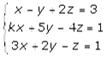1. We find the rank of the matrix of coefecientes.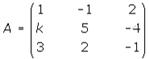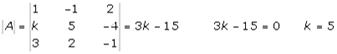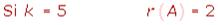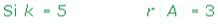2. We find the rank of the augmented matrix.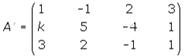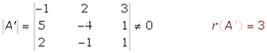3. We apply Rouche's theorem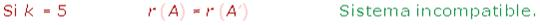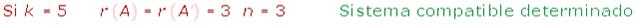4. We solve the system compatible determined by Cramer's rule (also can be solved by the Gauss).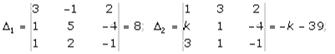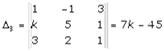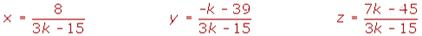Calculating the inverse matrix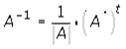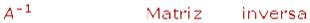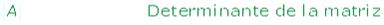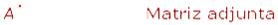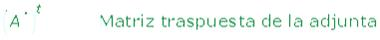Calculating the inverse matrix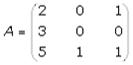1. We calculate the determinant of the matrix, where the determinant is null or the array will not reverse.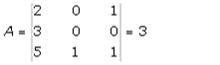2. We find the attached matrix, which is one in which every element is replaced his deputy po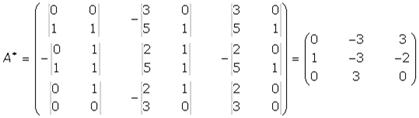> 3. We calculate the transpose of the matrix attached.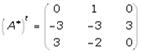4. The inverse matrix is equal to the inverse of the value of its determinant for the matrix transpose of the enclosed.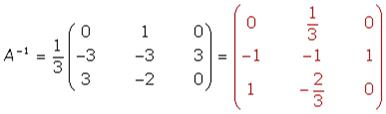Matrix equations formulas

1st case
X + B = C
X + B? B = C? B
X = C? B
2nd case
AX = C
If there is the inverse of A, | A |? 0
A -1 AX = A -1 C
IX = A -1 C
X = A -1 C
3rd case
XA = C
If there is the inverse of A, | A |? 0
AA -1 X = CA -1
C IX = A -1
X = CA -1
4th case
AX + BX = C
(A + B) X = C
(A + B) -1 (A + B) X = (A + B) -1 C
IX = (A + B) -1 C
X = (A + B) -1 C
Multiplication Arrays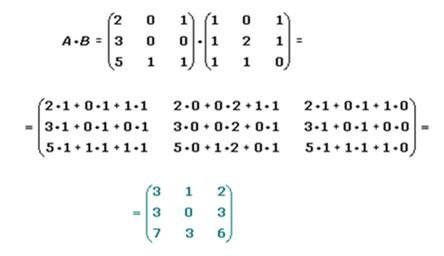Multiple rows of columns